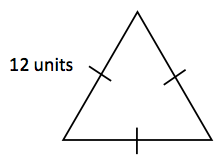### Home > INT2 > Chapter 7 > Lesson 7.1.2 > Problem7-32

7-32.

Calculate the area of an equilateral triangle with side length $12$ units. Show all work.

Sketch an image of the triangle similar to the one below.Remember the equation for the area of a triangle:

$A=\frac{1}{2}bh$

Draw in the height of the triangle. Since this is an equilateral triangle, the height will split the base in half.

Use Pythagorean Theorem or $30^{\circ}\text{-}60^{\circ}\text{-}90^{\circ}$ triangle pattern to calculate the height of the triangle.